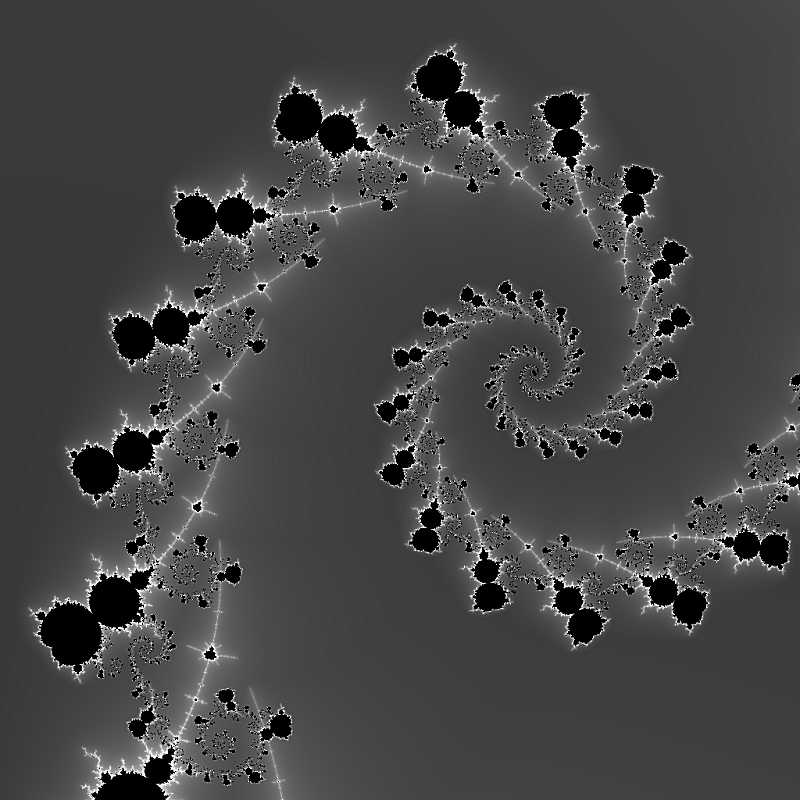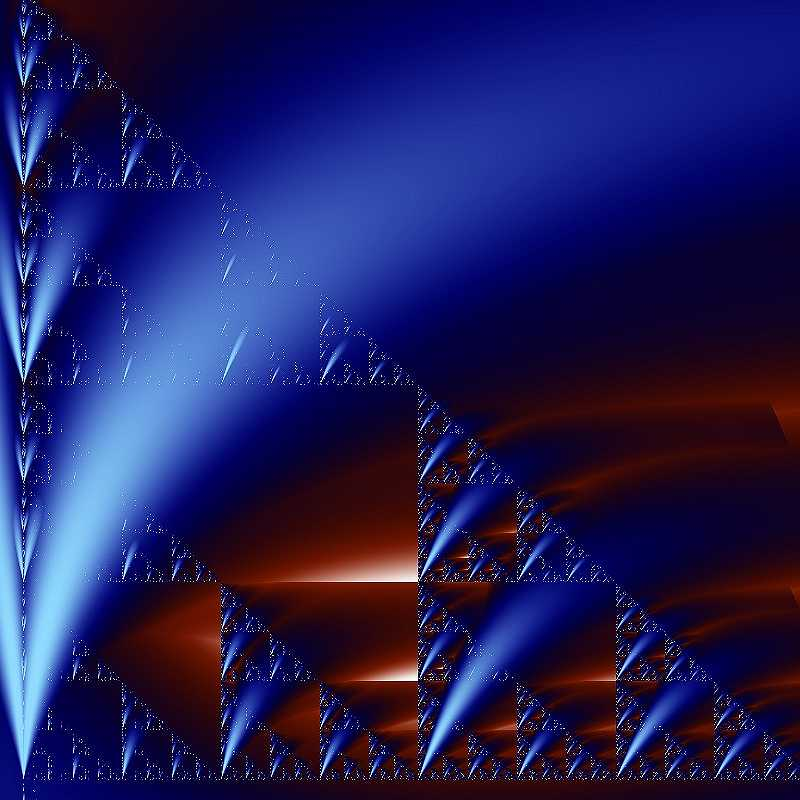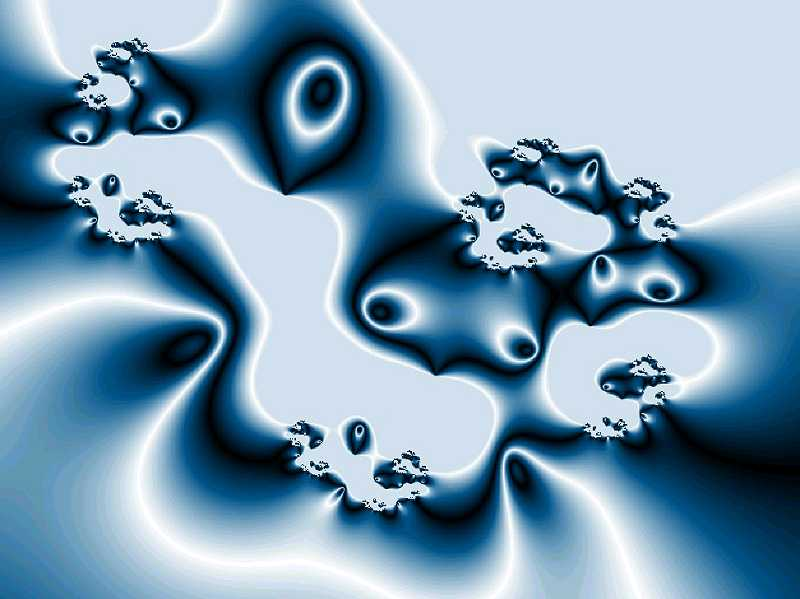Fractals and multi layer
coloring algorithms

Javier Barrallo and Santiago Sanchez

Mathematics, Physics and Computer Science

The University of the Basque Country

School of Architecture. Plaza Onati, 2.

20009 San Sebastián, SPAIN

Abstract

 The focus of exploration in new fractal techniques is shifting away from fractal formulas and towards coloring algorithms. This paper provides an overview of the coloring algorithms in popular use and how they can be combined and superposed. We call these multi layer fractals, and they are the source of some of the richest fractal imagery being produced today. In this way, even a limited collection of fractal coloring algorithms can be combined in almost endless ways.

1. Dynamical systems and fractals

Dynamical systems are a well-known branch of mathematics, but until the arrival of computers the sheer number of calculations involved made them impractical for real use. The computers ability to perform rapid calculations allows us to condense billions of calculations into results we can digest. Benoit Mandelbrot was the first one to use computers to produce graphical representations of dynamical systems in the complex plane, based on the quadratic formulas described by the French mathematician Gaston Julia at the beginning of the 20th century.

During the 1980s, fractal enthusiasts began exploring fractals for their artistic merit, not for their mathematical significance. While mathematics was the tool, the focus was art. As the fractal equation itself was the most obvious mathematical element, fractal artists experimented with new equations, introducing hundreds of different fractal types. By carefully choosing parameters to refine form, color, and location, these explorers introduced the concept of fractal art.

After 1995, few new major fractal types have been introduced. This is because the newest innovations in fractal art do not come from changing the fractal equation, but from new ways of coloring the results of those equations. As these coloring algorithms move from simple to complex, fractal artists are often returning to the simpler, classical fractal equations. With the increased flexibility these sophisticated algorithms provide, there is even more room for personal artistic expression.

2. Coloring algorithms

Every dynamical system produces a sequence of values z0, z1, z2 zn. Fractal images are created by producing one of these sequences for each pixel in the image; the coloring algorithm is what interprets this sequence to produce a final color.

Typically, the coloring algorithm produces a single value for each pixel. Since color is a three-dimensional space, this one-dimensional value must be expanded to produce a color image. The common method is to create a palette, a sequence of 3D color values; these are connected end-to-end and the coloring algorithm value is then used as a position along this multi-segmented line (the gradient). If the last palette color is connected to the first, a closed, segmented loop is formed and any real value from the coloring algorithm can be mapped to a defined color in the gradient. Gradients are normally linearly interpolated in RGB space (Red, Green, Blue), but they can also be interpolated in HSL space (Hue, Saturation, Lightness) and interpolated with spline curves instead of straight line segments.

The selection of the gradient is one of the most critical artistic choices in creating a good fractal image. Color selection can emphasize one part of a fractal image while de-emphasizing others. In extreme cases, two images with the same fractal parameters, but different color schemes will appear totally different.

Some coloring algorithms produce discrete values, while some produce continuous values. Discrete values will produce visible stepping when used as a gradient position; until recently this was not terribly important, as the restriction of 8-bit color displays introduced an element of color stepping in gradients anyway, and discrete coloring values were mapped to corresponding discrete color in the gradient. With the introduction of inexpensive 24-bit displays, algorithms which produce continuous values are becoming more important, as this permits interpolating along the color gradient to any color precision desired.

3. Fractal types

There are many different fractal types which are not covered when explaining coloring algorithms because they are either not common in fractal explorations or because they are not present in the popular fractal software packages.

But basically, we can classify fractal types in six main groups:

a) Fractals derived from standard geometry by using iterative transformations on an initial common figure like a straight line (the Cantor dust or the von Koch curve), a triangle (the Sierpinski triangle), or a cube (the Menger sponge). The first fractal figures invented near the end of the 19th and early 20th centuries belong to this group.

b) IFS (Iterated Function Systems). This is a type of fractal introduced by Michael Barnsley. The structure of these fractals is described by a set of affine (linear) functions that compute the transformations undergone by each point by homothety, translation, and rotation. The functions introduced into the system are selected randomly, but the final set is fixed and shows fractal structure.

c) Strange attractors. These sets can be considered as the representation of a chaotic movement (in no place and no time identical). These attractors are very complex and composed by a line of infinite length drawing tightly intertwined loops that never crosses its own trajectory.

d) Plasma fractals. Created with techniques like the fractional Brownian motion (fBm) or the midpoint displacement algorithm, these fractal type produce beautiful textures with fractal structure, like clouds, fire, stone, wood, etc. widely used in CAD programs. Skilled fractal artists love plasma to create textures or backgrounds in their images.

e) L-Systems, also called Lindenmayer systems, were not invented to create fractals but to model cellular growth and interactions. A L-System is a formal grammar that recursively applies its rules to an initial set. As a result, sometimes, a fractal structure is created.

f) Fractals created by the iteration of complex polynomials: perhaps the most famous fractals (Julia, Mandelbrot). Only this group of fractals has been widely experimented with different coloring algorithms by the fractal art community.

Many fractals sets can be considered subgroups from this types, for example fractal terrains are a three dimensional representation of a plasma fractal. Fractal music is the sonic representation of a chaotic attractor movement. Other fractals, like quaternionic or (recently) hypernionic fractals may be considered as an extension to higher dimensions of polynomial fractals iterated in the complex plane.

4. Fractal types and coloring algorithms

With the increasing significance of coloring algorithms it is surprising that there has been no attempt to use the variety of algorithms that are popping up with many of the fractals types described before. This paper may be considered as an initial attempt to combine the most important fractal types with different coloring algorithms.

The first step is the migration of fractal types a) - e) towards the last one f). This is because complex variable supports all the coloring algorithms and can work with all the fractal types applying minor transformations. Basically it is necessary the edition of the formulas to substitute the real variables corresponding to the X and Y axis with a single complex variable and to prepare the initial conditions under the formulas should be iterated. Then, the values used by the fractal types a) - e) are mapped into a discrete field of NxM pixels whose values are assigned to a complex variable which can be easily iterated.

As an example, Figures 1-6 show fractal types a) - f) as they are usually computed. Figures 7-12 show the same fractal types artistically interpreted using different coloring algorithms.

4. Multi layer fractals

A more significant technique involves combining techniques. We call these multi layer fractals, and they are the source of some of the richest fractal imagery being produced today. In this way, even a limited collection of fractal coloring algorithms can be combined in almost endless ways, with each combination becoming in effect an entirely new algorithm.

Figures 13-15 shows the three layers of the picture "The eyes of the beholders" using different algorithms, meanwhile figure 16 shows the final picture with the previous three layers merged together

References

 J. Barrallo, Fractal Geometry. 2nd Edition. Ed. Anaya Multimedia. Madrid 1992.  J. Barrallo & D. M. Jones, Coloring algorithms for dynamical systems in the complex plane, ISAMA 99 Proceedings, pp. 31-38. The University of the Basque Country, 1999.Figures 1-6: Fractal types represented in the standard way without coloring algorithms. From top left to bottom
down: a) Classic fractal, b) IFS, c) Strange attractor, d) Plasma fractal, e) L-System f) Complex polynomial.Figures 7-12: The same fractal formulas and parameters of figures 1-6 are repeated here, but
this time complex variable and coloring algorithms have been used for their calculation.Figures 13-16: "The eyes of the beholders". The picture is composed by three different images (top) using
the same formula and parameters but different color algorithms. The final image is the result of the superposition
of the layers containing the three previous images. This kind of image is known as multi layer fractal.

VisMathHOME